Latest Banking jobs   »   Quantitative Aptitude Quiz For SBI Clerk...

# Quantitative Aptitude Quiz For SBI Clerk Mains 2022- 11th December

Directions (1 – 5): Read the given information carefully and answer the questions.

There are 10 red balls and X yellow balls in a bag. When two balls are drawn from the bag without replacement, then probability of getting at least one yellow ball is  29/38. Veer invested Rs 100X in a scheme offering simple interest at 18% p.a. After 3 years, Veer gifted Y% of what he received in total from the scheme to Ayush which he distributed between Anurag & Amit in ratio (X + Y) : X respectively.  Amount received by Amit is Rs 110. Amit mixed Y lit of pure milk with 40 lit of water from which Deepak took Z lit of mixture, due to which Amit is left with (Y – X) lit of milk.

Q1. which of the following is true?

(a) X < Y < Z

(b) X < Y > Z

(c) X > Y > Z

(d) X > Y < Z

(e) X = Y = Z

Q2. If Veer has gifted all the amount he received from the scheme to Ayush and Ayush further distributed it in ratio of 7 : 4  to Anurag & Amit respectively, then how much Amit would have received more?

(a) Rs 320

(b) Rs 340

(c) Rs 220

(d) Rs 450

(e) Rs 420

Q3. What is difference between quantity of milk & water in the mixture Deepak has?

(a) 2 lit

(b) 4 lit

(c) 0 lit

(d) 6 lit

(e) 8 lit

Q4. If Amit replaced quantity of mixture taken by Deepak with equal quantity of milk, then what is ratio of milk to water in the mixture that Amit has after replacement?

(a) 59 : 31

(b) none of these

(c) 17 : 10

(d) 4 : 5

(e) 29 : 16

Q5. Quantity I: in how many ways can 3 yellow balls be picked from the bag, when 3 balls are drawn from the bag?

Quantity II: Numerical value of  XYZ/(X+Y+Z).

(a) Quantity I < Quantity II

(b) Quantity I = Quantity II or no relation

(c) Quantity I > Quantity II

(d) Quantity I ≥ Quantity II

(e) Quantity I ≤ Quantity II

Directions (6-9): Each of the following question is followed by two statements i.e. Statement I & II. You have to determine which statement (s) is/are sufficient/necessary to answer the question and mark answer accordingly.

(a) Only Statement I alone is sufficient

(b) Either statement I alone or II alone is sufficient

(c) Both statements together are not sufficient

(d) Only statement II alone is sufficient

(e) Both statements together are necessary

Q6. What is present age of Aman?

I: Aman’s age 5 years ago was equal to Veer’s age 2 years ago. Sum of present ages of Veer & Ayush is 49 years.

II: Shivam who is 4 years younger to Aman will be equal to present age of Ayush after 4 years.

Q7. by what percent mark up price is higher than cost price?

I: after allowing a discount of 50%, 10% profit is earned on selling price.

II: if 20% discount is offered then profit earned becomes equal to cost price.

Q8. how many red balls are in the bag, bag contains red, white, yellow and blue balls?

I: there are 12 yellow balls in the bag which are 20% more than white balls.

II: if a ball is drawn at random from the bag, then the probability of getting a blue ball is  1/10.

Q9. in how many days A alone can complete a piece of work?

I: B is 20% more efficient than A and C alone can complete the work in 10 days.

II: C is 10% less efficient than B.

Directions (10 -15): In each of these questions, two equations (I) and (II) are given. You have to solve both the equations and give answer

(a)   if x>y

(b)   if x≥y

(c)   if x<y

(d)   if x ≤y

(e)   if x = y or no relation can be established between x and y.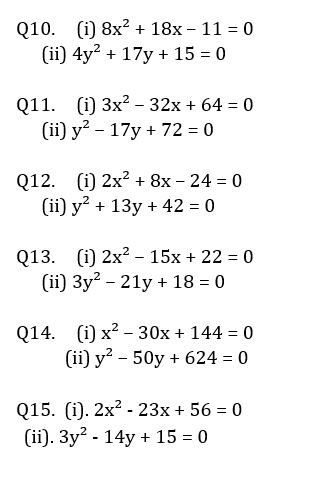Solutions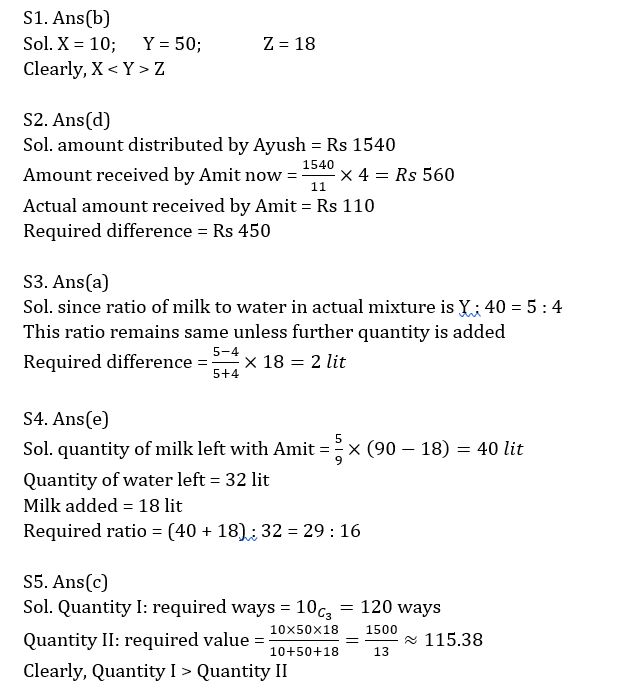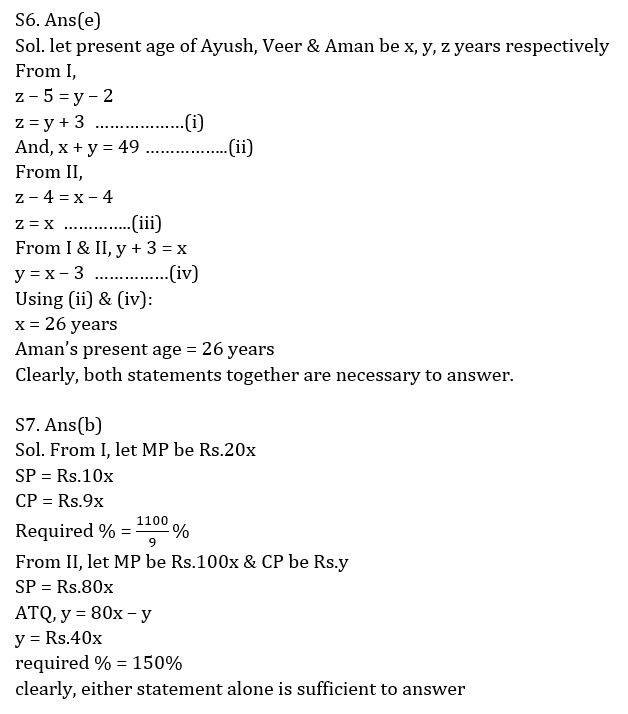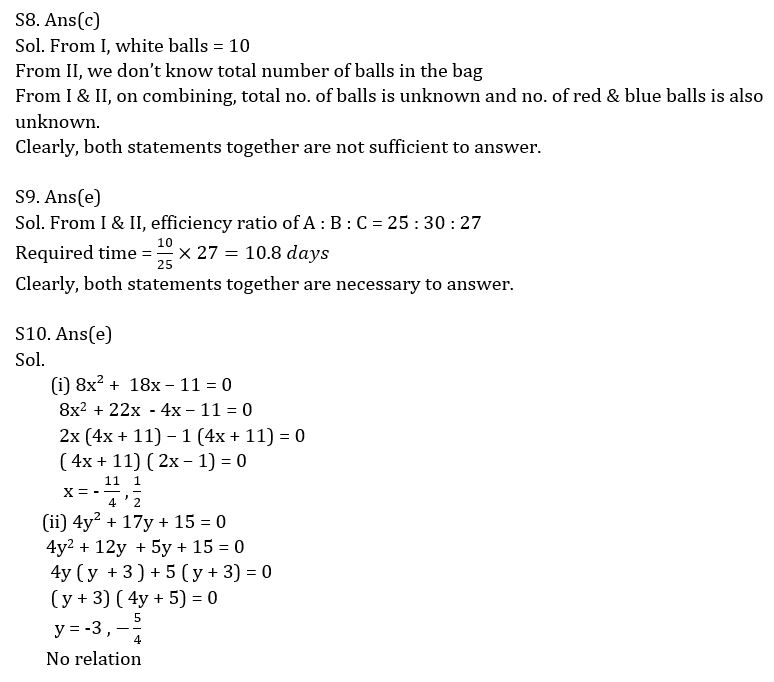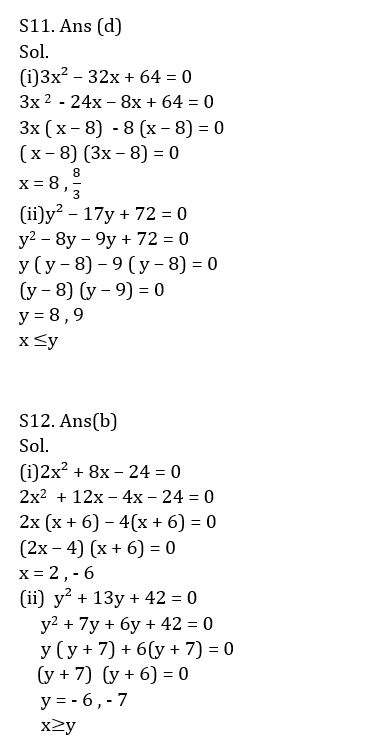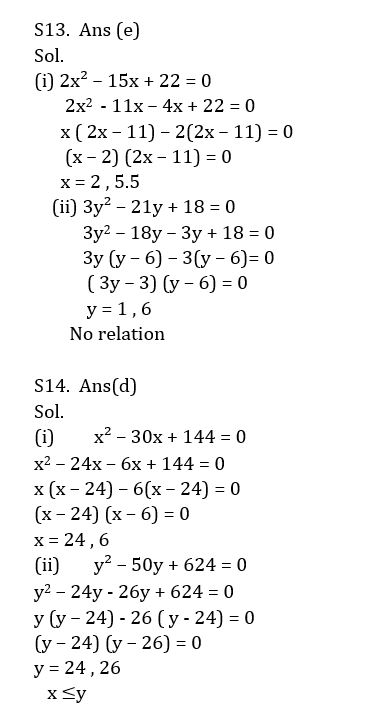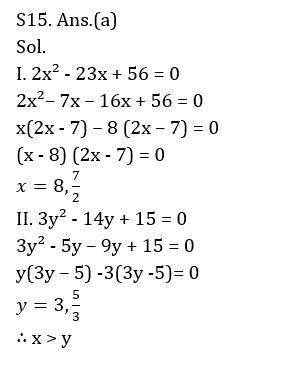#### Congratulations!Union Budget 2023-24: Free PDF